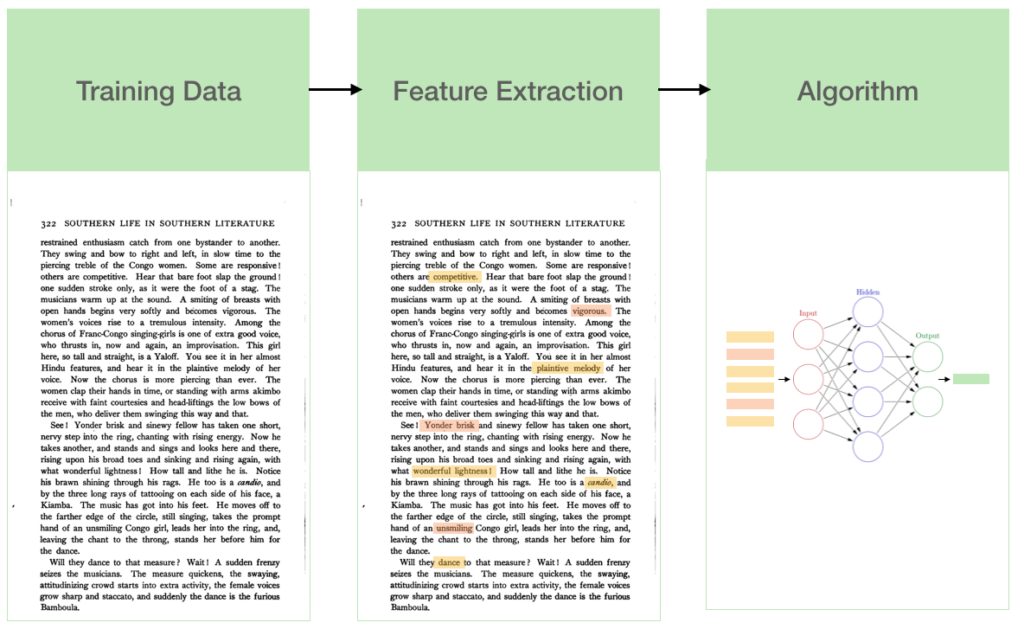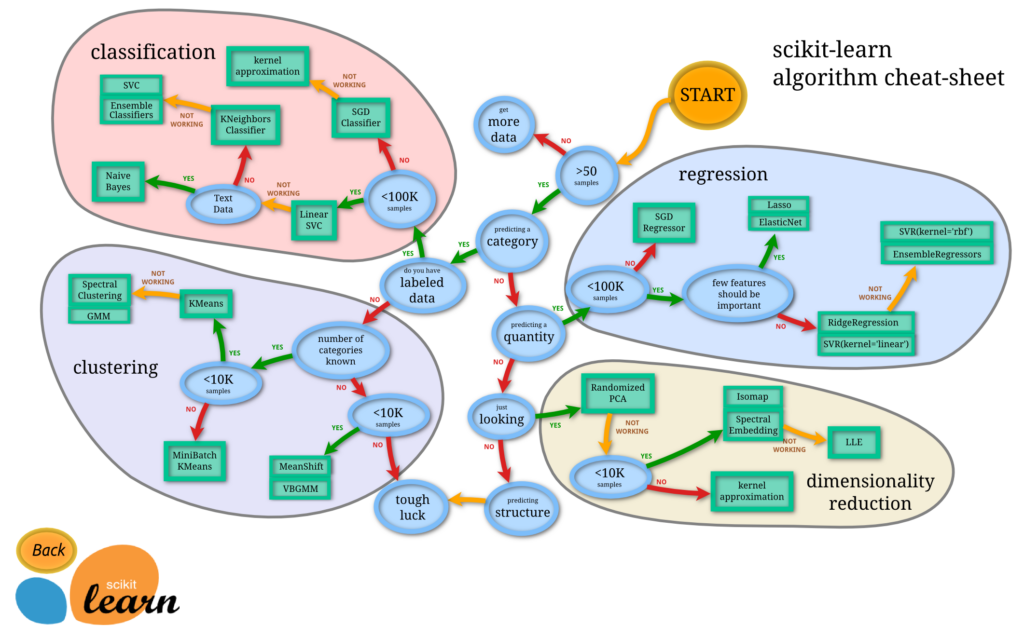## Sentiment Analysis

In this video we build a sentiment analysis classfier on a small twitter dataset. We introduce the scikit learn library.

Project

Check your understanding by building a more advanced text classifier by going to https://github.com/lukas/ml-class/tree/master/projects/5-sentiment-analysis

Topics

• Introduction to scikit and pandas
• Feature extraction and “bag of words”
• Transforming data
• Choosing an algorithm for text classification
• Limitations of text classification algorithms

## Sentiment Analysis

In this tutorial, we are going to build a model that classifies tweets about a brand as having either a positive or negative sentiment, and extract the topic of the tweet. This is a really common scenario – every major consumer company uses machine learning to do this.The first thing we need to do this is training data.  Examples of positive and negative tweets about my brand.  Without training data, machine learning almost never works.

Go to the directory scikit and open up tweets.csv in a text editor, or excel or any other program. This is a raw file with a few thousand tweets of labeled data called tweets.csv.  These are tweets about Apple products taken at south by southwest and humans at Figure Eight went through and labeled them as positive negative neutral or can’t tell.

## Feature Extraction

The first thing that we want to do with text training data is feature extraction. As you may recall from the first tutorial, machine learning algorithms have a very simple and constrained API. They take in a fixed length set of numbers and output a fixed length set of numbers. They don’t generally take text, audio or images as input, so we need to turn each piece of text into a fixed length set of numbers.A surprisingly powerful way to do this is called bag of words. This means that every tweet is converted into a vector, where each column represents a word. The value for the vector in each column is the number of time that word appears in the tweet. The total number of unique words used in all of your training data is known as the vocabulary. Therefore, the number of columns in the vector will be equal to the size of the vocabulary – in this case 9706. For each tweet, most of the columns will be 0.

## Code

Open up the directory scikit in the ml-class folder. In here you will find a csv file with tweets about Apple products, classified by Figure Eight. We want to build a neural network to classify the sentiment of these tweets into positive and negative.

The first thing we need to do is load the data into python. Open up load-data.py. Here we use the pandas and numpy library to put the tweets into a dataframe, with a column reflecting the emotion.

Now we perform feature extraction. Open up feature-extraction-1.py. The first 5 lines are the same as load-data and just load in our tweets. We then import the scikit learn CountVectorizer. This converts our text to a bag of words. When we run this program however, we see that an error is thrown. Take a subset of your data and run it again. Can you figure out where the bug is? (Hint, open up tweets.csv)

The error is that there is a blank entry in tweet 8. Scikit will not accept blank inputs. Let’s open up feature-extraction-2.py to see how to deal with this.feature-extraction-2.py

Now, on line 11, we set fixed text equal to text, with a pandas function that removes all null entries. We do the same for target. This type of data cleanup is super important – don’t do it manually, do it in the code. Now we have set up the transformation, let’s actually do the transformation in feature-extraction-3.py. This code is the same as feature-extraction-2.py with the following code added at the end:

counts = count_vect.transform(fixed_text)

This creates a sparse matrix with our vocabulary. Now we are ready to build our algorithm.

## Choosing an algorithm

Ok let’s build a classifier. But first – how do we choose an algorithm? There are a number of popular methods for different use cases.

The choice of algorithm is tough for beginners and argued about by experts but I think a great rule of thumb is to use this excellent flowchart made by scikit.If you generally walk through this flow chart, you will get to a reasonable algorithm. Let’s start at the top. We have greater than 50 samples of data, and are predicting a category. We have labelled data, and less than 100,000 samples (we have around 10,000). It then recommends a linear SVC. Linear SVC for this case doesn’t actually work very well, so if we follow the ‘not working arrow’, we get to Naive Bayes.

Naive Bayes is a simple algorithm that generally does work really well and it works very fast. Let’s try it out on our dataset.

Open up classifier.py. From scikit learn we just import the multinomial naive bayes algorithm using the following code:

from sklearn.naive_bayes import MultinomialNB

nb = MultinomialNB()
nb.fit(counts, target)

Counts is bag of words which records the frequency of words occurring in tweets, and target is the sentiment we are trying to classify. Play around with classifier.py to see how the model works on some sample tweets.